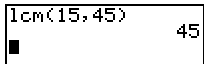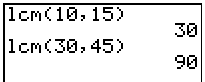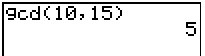LCM and GCF

Finding the Least Common Multiple (LCM):

 LCM is found under MATH → NUM, arrow down to #8:  lcm( From the home screen, choose lcm(  followed by two numbers separated by a comma.NOTE:  lcm( accepts non-negative integers only and works with only two entries at a time. Example:   Find the LCM for 10, 15, and 45. Since LCM can only accept two entries at a time, find the LCM of the first two values, and then find the LCM of your answer and the third value.Finding the Greatest Common Factor (GCF):

 NOTE:  The calculator refers to the GCF as the GCD, greatest common divisor. GCD is found under MATH → NUM, arrow down to #9:  gcd( From the home screen, choose gcd(  followed by two numbers separated by a comma.NOTE:  gcd( accepts non-negative integers only and works with only two entries at a time. Example:   Find the GCF (or GCD) for 12, 24, and 32. Since GCD can only accept two entries at a time, find the GCD of the first two values, and then find the GCD of your answer and the third value.Finding Your Way Around TABLE of  CONTENTS